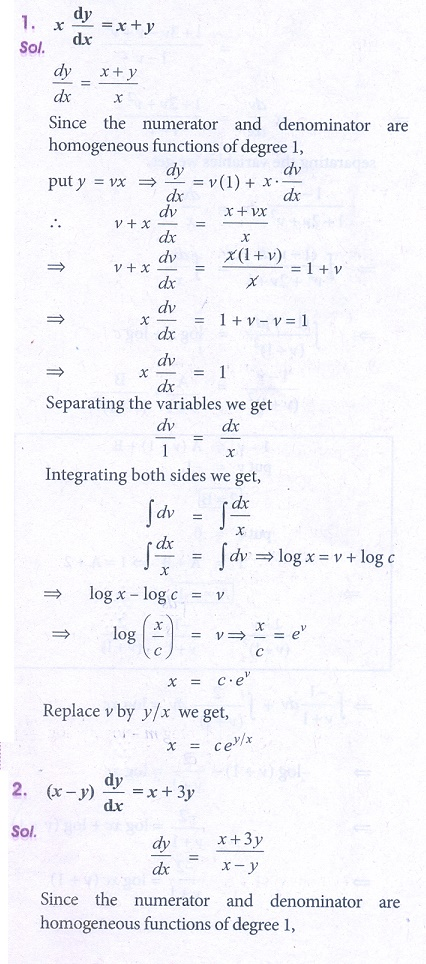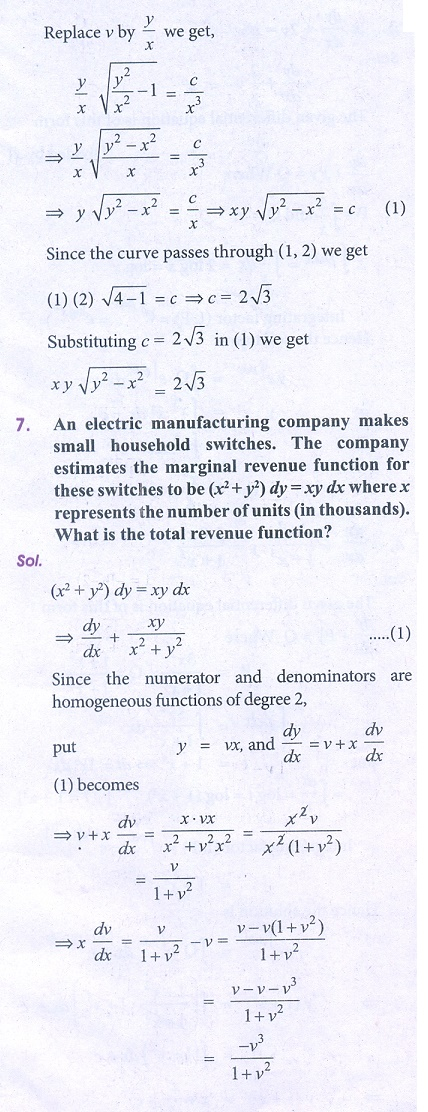Home | | Business Maths 12th Std | Exercise 4.3: Homogeneous Differential Equations

# Exercise 4.3: Homogeneous Differential Equations

Book back answers and solution for Exercise questions - Maths: Differential Equations: Homogeneous Differential Equations

Exercise 4.3

Solve the following homogeneous differential equations.6. The slope of the tangent to a curve at any point (x, y) on it is given by (y3  2 yx2 )dx +(2xy2  x3 ) dy = 0 and the curve passes through (1, 2). Find the equation of the curve.

7. An electric manufacturing company makes small household switches. The company estimates the marginal revenue function for these switches to be (x2 + y2 )dy = xydx where x represents the number of units (in thousands). What is the total revenue function?